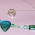## Wednesday, July 11, 2007

### Solution to the Question on Two Dimensional Motion

In the post dated 10th July 2007, a questions on two dimensional motion was given without solution. The question with solution is given below as promised in the post:

A steel sphere A projected up with a velocity of 10 ms–1 at an angle of 60º with the horizontal, collides elastically with an identical steel sphere B located at the highest point of the trajectory of A. The sphere B is the bob of a simple pendulum. After the collision, if the sphere B just moves along a vertical circle, what is the length of the pendulum? ( Take g = 10 ms–2)

(a) 50 cm (b) 55 cm (c) 60 cm (d) 65 cm (e) 70 cm

At the highest point of the trajectory, the velocity of the projectile is horizontal and is equal to ucosθ = 10 cos 60° = 5 ms–1. Since the collision is elastic and the spheres are identical, the sphere A transfers its entire momentum to sphere B. Sphere B therefore moves with the same horizontal velocity of 5 ms–1.

If the sphere is to just move along a vertical circle, the minimum velocity required at the lowest point of the circle is (5gR) where ‘R’ is the radius of the circle (which is the length of the pendulum here). Therefore, we have

(5gR) = 5 ms–1.

On substituting for the acceleration due to gravity, g (=10 ms–1), R = 0.5 m = 50 cm.

Now, consider the following MCQ:

A ball rolling along a horizontal floor with a velocity of 7 ms–1 reaches the edge of a long stair case from where it moves down as a projectile. If the width and the height of the steps of the stair case are 25 cm and 10 cm respectively, the ball will strike the edge of the nth step from the top, where n is equal to

(a) 10 (b) 12 (c) 14 (d) 16 (e) 18

Since the horizontal velocity is unchanged throughout the trajectory of the ball, we have

7 t = 0.25 n where ‘t’ is the time taken to hit the edge of the nth step after leaving the top of the stair case.

Therefore, t = 0.25 n / 7

The vertical fall of the ball during this time is ½ g t2 and we have

½ g t2 = 0.1 n

Substituting for t and g, ½ ×9.8×0.0625 n2/49 = 0.1n

Therefore, n = (49×0.1)/(4.9×0.0625) =16

1.2.